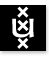Advanced Topics in Set Theory 2004/2005; 1st Semester Institute for Logic, Language & Computation Universiteit van Amsterdam
Instructor: Dr Benedikt Löwe
Vakcode: MolATST6
Time: Thursday 17-19
Place: P.017
Course language: English
Teaching Assistant: Brian Semmes
Intended Audience: M.Sc. students of Logic and Mathematics
Prerequisites: This course assumes knowledge comparable to the course "Axiomatic Set Theory".

In this course, we shall discuss connections between set theory and game theory. We investigate infinite perfect information games, their connection to the Axiom of Choice, the Mycielski-Steinhaus Axiom of Determinacy and its consequences for infinitary combinatorics.

Literature: Preprint version of Alessandro Andretta's book on Descriptive Set Theory. The distribution of copies is organized by Samson de Jager.

Classes:

• First lecture (September 9th, 2004). The Axiom of Choice. Restricted versions of AC. The Axiom of Dependent Choices. Trees and wellfoundedness. (Section 1)
Homework (due Sep 16, 2004). Exercise 1.13 (p.8), Exercise 1.22 (i) (p.13).
• Second lecture (September 16th, 2004). Assigning ordinals to wellfounded trees. A ZF-surjection from the reals onto omega1. Topology of Baire space. Continuous and Lipschitz functions. Combinatorial characterization. Trees and closed sets (no proofs). Cantor-Bendixson (no proof). sigma-algebras and Borel sets. (Sections 1 to 3)
Homework (due Sep 23, 2004). Exercise 2.2 (i), (ii), (iv), Exercise 2.3, Exercise 2.7 (i), (ii), Exercise 2.20 (ii).
• Third lecture (September 23rd, 2004). Borel classes. AC and the Borel sets. The height of the Borel hierarchy. Pointclasses. Universal sets. Analytic sets. (Sections 3 and 4)
Homework (due Sep 30, 2004). Exercise 3.5 (i), Exercise 3.9 (iv), (v).
• Fourth lecture (September 30th, 2004). Analytic sets. Projective sets. The projective hierarchy. The language of second-order number theory. Effective descriptive set theory.
• (October 7th, 2004). No lecture.
• Fifth lecture (October 14th, 2004). Borel separation theorem (without proof). Codes for wellorders and wellfounded trees. LO, WO and WF. WF is Pi11-complete. Boundedness Lemma. Infinite two-player games: Definitions and Strategies. (Sections 4B and 8A.)
Homework (due October 21, 2004). Exercises 4.44 (ii), 8.1, 8.2.
• Sixth lecture (October 21st, 2004). Two new notions of strategies and their equivalence. Determinacy. ADX. Equivalence of AD2 and ADN. The existence of a well-ordering of the reals implies that AD is false. AD implies AComega(R).
Homework (due Nov 5, 2004). Exercises 8.3, 8.4 (i), 8.5 (iii), 8.66.
• (October 28th, 2004). No lecture: Exam week.
• Seventh lecture (November 4, 2004). Gale-Stewart Theorem (Open Determinacy). Long games. Determinacy of games of length omega+n. Choice and games of length omega+omega. AD and the perfect set property. Existence of choice functions for WO and the perfect set property. Inconsistency of ADomega1 and ADP(R).
Homework (due Nov 11, 2004). PDF-File
• Eighth lecture (November 11, 2004). AD implies that there is no uncountable sequence of distinct reals. Inconsistency of games of length omega1. Continuously coded games. The asymmetric game. AD implies PSP. Det(Gamma) implies PSP(Gamma) for boldface pointclasses Gamma. The constructible hierarchy L(x) for a real x. PSP implies that omega1L(x) is countable.
Homework (due Nov 18, 2004). Exercises 8.31 (ii), 8.71, 9.3, and 10.2 (iv).
• Ninth lecture (November 18th, 2004). AD implies that omega1 is inaccessible in L. Measurable cardinals. The club filter and stationary sets. The club filter on omega2 is not an ultrafilter. Definition of a nonprincipal ultrafilter on N from an omega1-incomplete ultrafilter.
Homework (due Dec 2, 2004). PDF-File
• (November 25th, 2004). No lecture.
• Tenth lecture (December 2nd, 2004). AD implies that every ultrafilter is omega1-complete. Boundedness and Solovay games. AD implies that omega1 is a measurable cardinal: Solovay's proof. The Martin filter. Martin's proof.
Homework (due Dec 9, 2004). PDF-File
• Eleventh lecture (December 9th, 2004). Lipschitz and Wadge reducibility. Wadge's Lemma. Selfdual and nonselfdual degrees, examples. The Wadge jump. Wadge's Lemma and the perfect set property.
Homework (due Dec 16th, 2004). Exercises 11.6, 11.21, 11.23.
• Twelfth lecture.
• (December 23rd, 2004). No lecture: Exam week.

Last update : December 9th, 2004